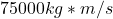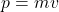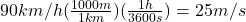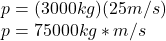## (0) Calculate the momentum of the vehicle when it moves at the maximum speed of 90 km/h with load of 3000 kg ​

Question

(0) Calculate the momentum of the vehicle when it moves at the maximum speed of 90 km/h with load of 3000 kg

in progress 0
5 months 2021-09-05T00:40:14+00:00 1 Answers 3 views 0

1. Answer:Explanation:where;

p = momentum

m = mass = 3000kg

v = velocity = 25m/s

————————————————————————————————–

The first thing we need to do is convert 90km/h to m/s. Our conversion factors here are: 1km = 1000m and 1h = 3600sPlug your values in the formula: